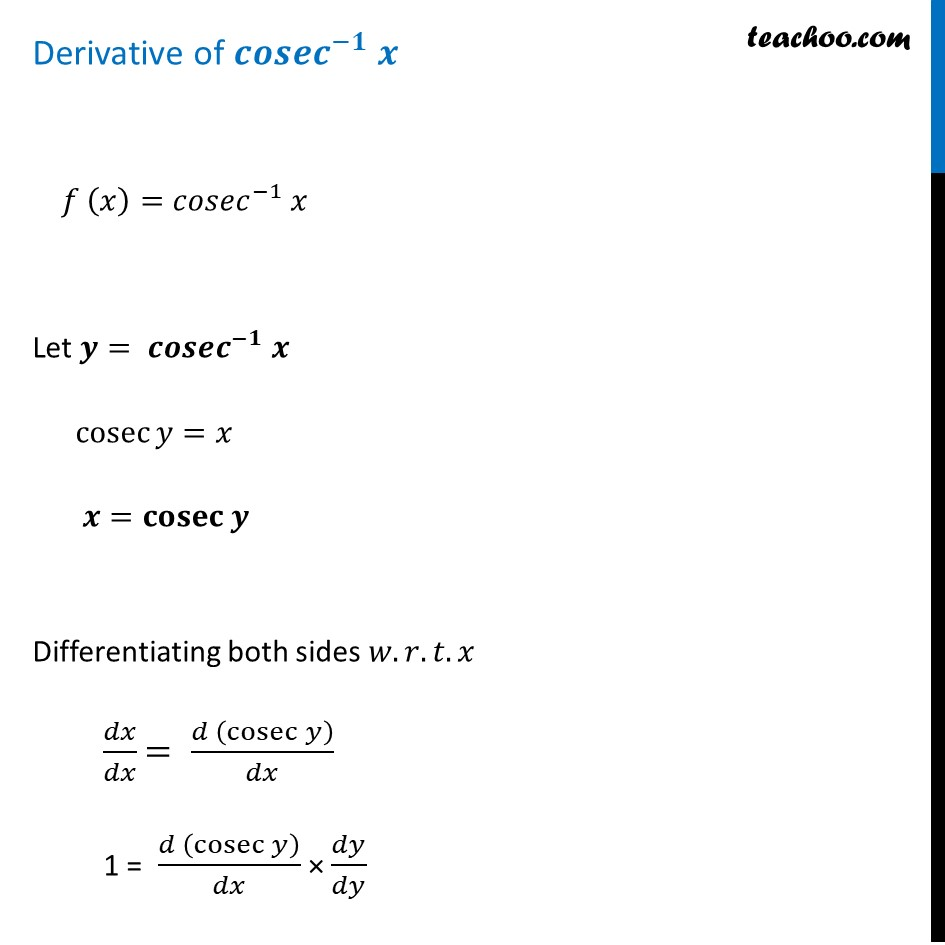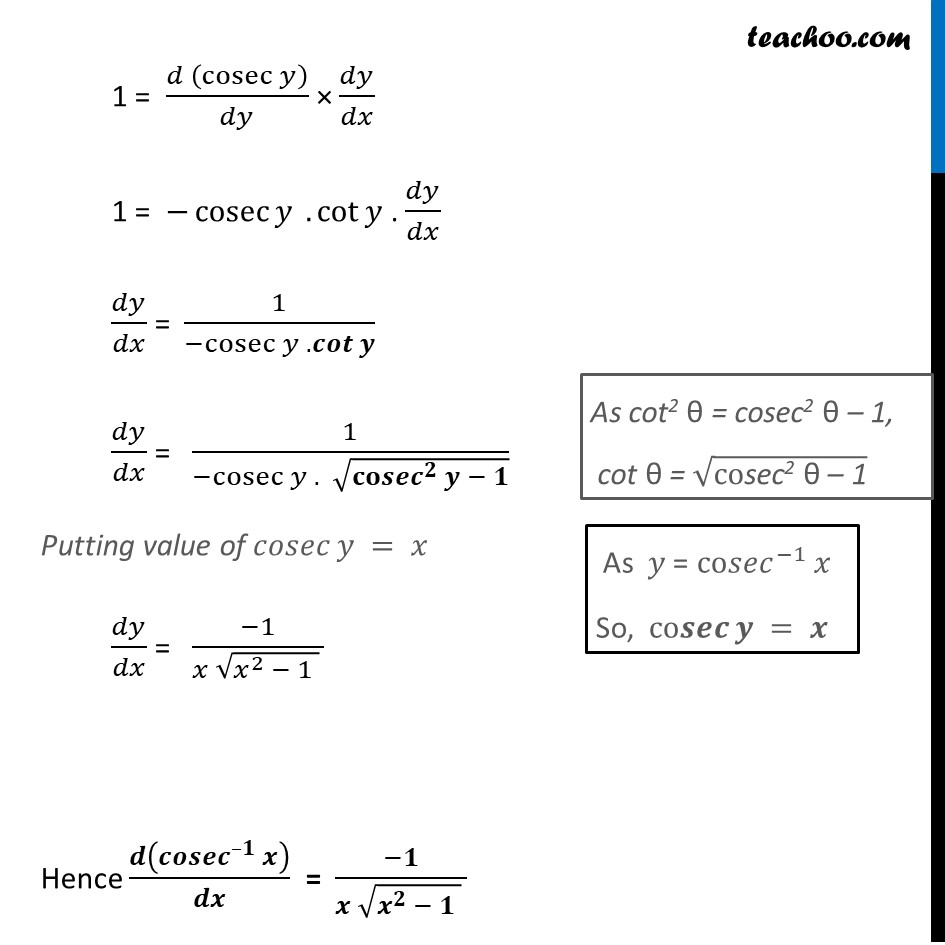Finding derivative of Inverse trigonometric functions

Chapter 5 Class 12 Continuity and Differentiability
Concept wiseLearn in your speed, with individual attention - Teachoo Maths 1-on-1 Class

### Transcript

Derivative of 〖𝒄𝒐𝒔𝒆𝒄〗^(−𝟏) 𝒙 𝑓 (𝑥)=〖𝑐𝑜𝑠𝑒𝑐〗^(−1) 𝑥 Let 𝒚= 〖𝒄𝒐𝒔𝒆𝒄〗^(−𝟏) 𝒙 cosec⁡〖𝑦=𝑥〗 𝒙=𝐜𝐨𝐬𝐞𝐜⁡〖𝒚 〗 Differentiating both sides 𝑤.𝑟.𝑡.𝑥 𝑑𝑥/𝑑𝑥 = (𝑑 (cosec⁡𝑦 ))/𝑑𝑥 1 = (𝑑 (cosec⁡𝑦 ))/𝑑𝑥 × 𝑑𝑦/𝑑𝑦 1 = (𝑑 (cosec⁡𝑦 ))/𝑑𝑦 × 𝑑𝑦/𝑑𝑥 1 = −cosec⁡𝑦 .cot⁡𝑦 . 𝑑𝑦/𝑑𝑥 𝑑𝑦/𝑑𝑥 = 1/(〖−cosec〗⁡𝑦 .𝒄𝒐𝒕⁡𝒚 ) 𝑑𝑦/𝑑𝑥 = 1/(〖−cosec〗⁡𝑦 . √(〖𝐜𝐨𝒔𝒆𝒄〗^𝟐⁡𝒚 − 𝟏)) Putting value of 𝑐𝑜𝑠𝑒𝑐⁡𝑦 = 𝑥 𝑑𝑦/𝑑𝑥 = (−1)/(𝑥 √(𝑥^2 − 1 ) ) Hence 𝒅(〖𝒄𝒐𝒔𝒆𝒄〗^(–𝟏) 𝒙)/𝒅𝒙 = (−𝟏)/(𝒙 √(𝒙^𝟐 − 𝟏 ) ) As cot2 θ = cosec2 θ – 1, cot θ = √("cosec2 θ – 1" ) As 𝑦 = co〖𝑠𝑒𝑐〗^(−1) 𝑥 So, co𝒔𝒆𝒄⁡𝒚 = 𝒙